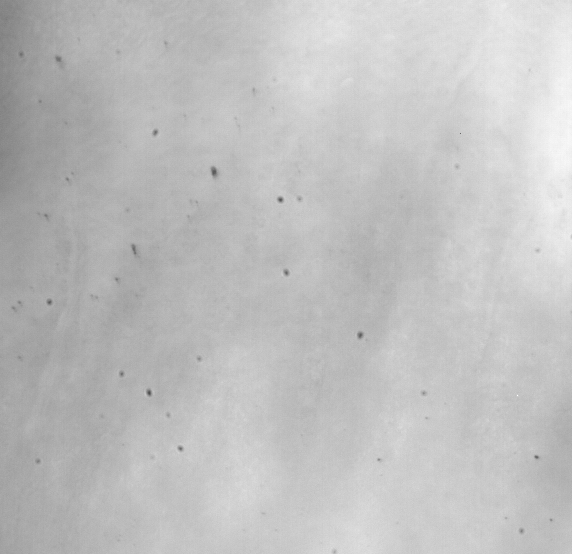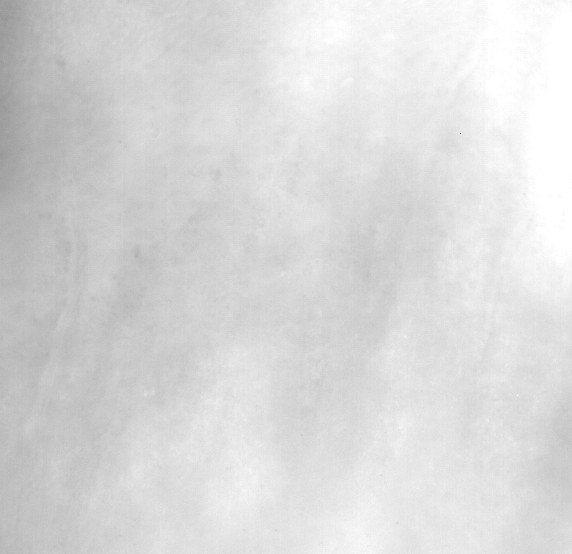## Problem:

It can happen that dust will stick on the reference mirror leading to dark spot on direct images, as visible on the following movie (palissade of voigt in a human in vivo-cornea, acquired by my fellow colleague Slava Mazlin):## How to remove the dust:

The first step is to threshold each frame. Because the lighting is not homogeneous I used an adaptive threshold where the threshold value is computed by taking into account the vicinity of the pixel (here $21\times21$ pixels). Then I filter the wrong detection based on the size by using morphological filters (typically it is single pixels so it is very easy to remove). Then the idea is to reconstruct the signal that was covered by the dust, which is called inpainting. To do that I propagate the information on the edge of each detected dust using Navier-Stokes equation, and tadaaa:Here is the code I used to filter the movie above (you need numpy, scikit-image and openCV to run it):

import numpy as np
from skimage.filters import threshold_local
from skimage.morphology import opening, dilation, diamond
from skimage.external.tifffile import TiffWriter
import cv2

def save_as_tiff(data, filename, resolution=(0.22,0.22)):
with TiffWriter(filename+'.tif', imagej=True) as tif:
for i in range(data.shape):
tif.save(np.squeeze(data[i]), compress=0, resolution=resolution)

if 'raw' not in locals():
raw_mean = np.sum(raw, axis =0)

# Build a mask by thresholding
t = (raw_mean > threshold_local(raw_mean, block_size=21, offset=1000)).astype('uint8')
t = 1 - t # Inverse it
t = opening(t, selem=diamond(1)) # Remove small detections
t = dilation(t, selem=diamond(1)) # Make the detected area wider

# Inpaint image using Navier-Stokes equation
raw_denoised = np.zeros(raw.shape)
for i in range(raw.shape):
raw_denoised[i] = cv2.inpaint(raw[i], t, 5, cv2.INPAINT_NS)

# Save results
save_as_tiff(raw_denoised.astype('uint16'), 'denoised', resolution=(1,1))